#### Euler method

For most systems, the integration must be performed numerically. A system simulator based on numerical integration can be constructed by breaking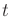into smaller intervals and iterating classical methods for computing numerical solutions to differential equations. The Euler method is the simplest of these methods. Let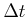denote a small time interval over which the approximation will be made. This can be considered as an internal parameter of the system simulator. In practice, thisis usually much smaller than theused in the discrete-time model of Section 14.2.2. Suppose that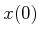and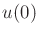are given and the task is to estimate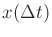.

By performing integration over time, the state transition equation can be used to determine the state after some fixed amount of timehas passed. For example, ifis given and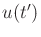is known over the interval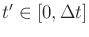, then the state at timecan be determined as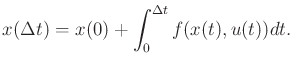(14.14)

The integral cannot be evaluated directly because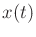appears in the integrand and is unknown for time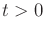.

Using the fact that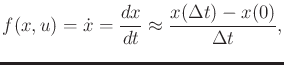(14.15)

solving foryields the classic Euler integration method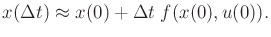(14.16)

The approximation error depends on how quicklychanges over time and on the length of the interval. If the planning algorithm applies a motion primitive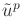, it gives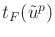as the time input, and the system simulator may subdivide the time interval to maintain higher accuracy. This allows the developer of the planning algorithm to ignore numerical accuracy issues.

Steven M LaValle 2020-08-14